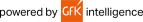# Texas Instruments TI-83 Plus Graphing Calculator - 10 Unit Classroom PackMSRP: 1499.99
Savings: \$393.14

Quantity

IN STOCK

Product Highlights
The TI-83 Plus is an easy-to-use graphing calculator for math and science that lets students graph and compare functions, as well as perform data plotting and analysis. Its FLASH ROM memory allows ... More

Is This Item Right For Me? - Ask Our ExpertsCalifornia Residents:

### Product Description

The TI-83 Plus is an easy-to-use graphing calculator for math and science that lets students graph and compare functions, as well as perform data plotting and analysis. Its FLASH ROM memory allows students to update and add software applications (Apps).### Specifications

General Information
Manufacturer Texas Instruments, Inc
Brand Name Texas Instruments
Product Model TI-83 Plus
Product Type Graphing Calculator
Technical Information
Functions
• Rectangular functions
• Parametric expression
• Polar expression
• Recursively-defined sequences
• Graphing functions defined, saved, graphed, and analyzed at one time.
• Sequence graphing mode shows time series plot, cobweb/ stair-step plot, and phase plots
• Interactive analysis of function values, roots,maximums, minimums, integrals, and derivatives
• Matrix operations: Inverse, determinant, transpose, augment, reduced row echelon form, and elementary row operations
• Convert matrices to lists and vice-versa
• List-based one- and two-variable statistical analysis: Logistic, sinusoidal, median-median, linear, logarithmic, exponential, power, quadratic polynomial, cubic polynomial, and quadratic polynomial regression models
• Statistical plot definitions for scatter plots, xy-line plots, histograms, regular and modified box-and-whisker plots, and normal probability plots
• Advanced statistics features: Hypothesis testing functions, confidence interval functions, and one-way analysis of variance
• Probability distribution function: Normal, Student-t, Chi-square, Binomial, and Poisson
• Business function: Time-Value-of-Money (TVM), cash flows, and amortization. Full screen interactive editor for solving TVM problems
• Memory
Standard Memory
• 160 KB Flash
• 24 KB RAM
• Display & Graphics
Display Screen 8 Line x 16 Character - LCD
Battery Information
Power Source Battery
Miscellaneous
Package Contents
• TI-83 Plus Teacher's Kit Graphic Calculator
• 3 x Guidebooks
• A Classroom Poster

Package Quantity: 10 Pieces### Customers Who Bought This Item Also Viewed